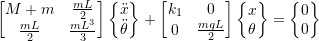### Review of single and multi-degree of freedom (mdof) systems: Stiffness and Flexibility Influence Coefficients

We can use the Newtonian approach to find the stiffness matrix and equations of motion. However, we can also use influence coefficients for both the mass and stiffness matrices.

Consider the definition of stiffness and flexibility coefficients:

## S.I.C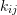is the force (moment) required at coordinateto maintain a unit linear (angular) displacement at coordinate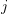with all other coordinates held fixed at zero

## F.I.C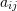is the linear (angular) displacement at coordinatedue to a unit force (moment) applied at coordinatewith all other coordinates free to move.

NOTE: All forces (moments) and displacements (rotations) are taken to be applied in the positive direction. The sign of the resulting forces (moments) or displacements (rotations) are determined to be positive or negative.

Also note that: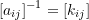## Example

###### Stiffness

Therefore: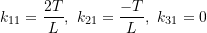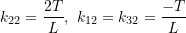Therefore: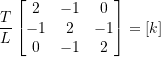###### Flexibilty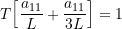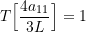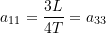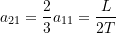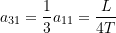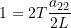Therefore: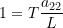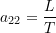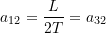Thus: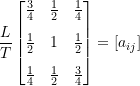Therefore: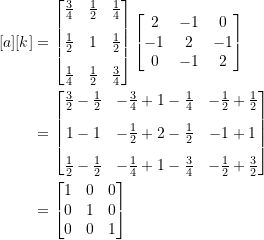As an example of MDOF, consider a general planar situation which can be specialized to different industrial situations. Many of these problems can be initially modelled as SDOF ones, and then MDOF analysis will allow interpretations of the simplifying assumptions made.

NOTE: While the body shown is a rectangular shape, it can be any rigid body with a massand moment of inertia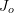about an axis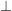to the plane through the center of gravity. The horizontal stiffness components, designated by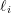are most often just the lateral stiffness of the vertically mounted spring. For real springs, the lateral spring may be hard to find in the literature. For coil springs, it is often in the range of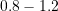times the longitudinal value.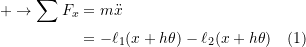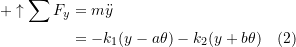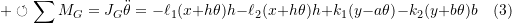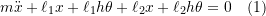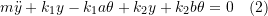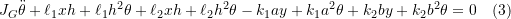In matrix form: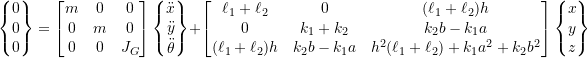Part 2

Now find the stiffness matrix using influence coefficients.

First consider a unit displacement in thedirection only: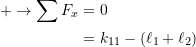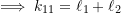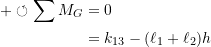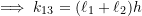For the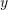direction unit displacement: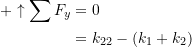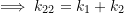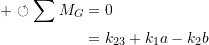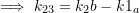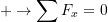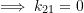Assuming all summations fordirection,direction and moment equal to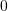: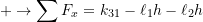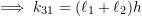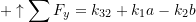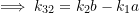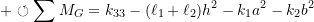Therefore: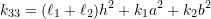This yields the same stiffness matrix.

As long as we use the motions about the center of gravity then the inertia(mass) matrix is just the mass on the mass moment of inertia about the center of gravity. If we use the coordinates to decide the motion that is not related to the absolute motion of the center of gravity then the stiffness matrix may not be symmetric and the mass matrix will not be diagonal.

#### Inertia Influence Coefficients

The elements of the mass matrix,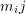can be determined through the use of inertia influence coefficients. They are defined as the set of impulses applied at the points (representing the coordinate directions)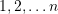respectively to produce a unit velocity at pointand zero velocity at every other point.

NOTE: If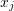denotes an angular coordinate then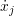represents an angular velocity

Thus for a multi-degree of freedom system, the total impulses at pointsis: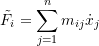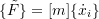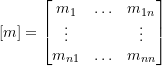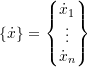To find the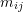1. Assume that a set of impulses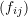are applied at all points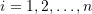as to produce a unit velocity at pointonly(with zero velocity in all the after dierections(coordinates)). By definition the set of impulses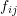denote the influence inertia coefficients2. Repeat the procedure for each point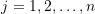.
###### Example

First apply unit velocity toonly: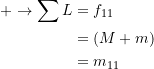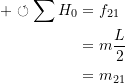Apply unit velocity(angular) to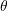only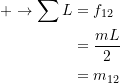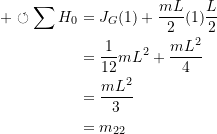Therefore: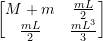Now find the stiffness matrix

Apply unit deflection toonly:

Therefore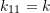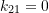Apply unit deflection toonly: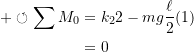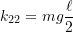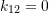Therefore: